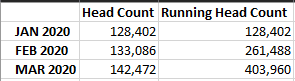cancel
Showing results for
Did you mean:Frequent Visitor

## Optimize Running Total

Hi All,

I'm currently running the following DAX code for a Running Total based on a calculated measure. I'm looking to optimise the below DAX code?

VAR _Date = MAX ('Fact_Table'[Date])
VAR _Date11 = EOMONTH (_Date, -11)
VAR _Date10 = EOMONTH (_Date, -10)
VAR _Date9 = EOMONTH (_Date, -9)

FILTER(
ALL('Fact_Table'), 'Fact_Table'[Date] = _Date11)
)

FILTER(
ALL('Fact_Table'), 'Fact_Table'[Date] = _Date10)
)

FILTER(
ALL('Fact_Table'), 'Fact_Table'[Date] = _Date9)
)

RETURN _Value11 + _Value10 + _Value9 + [Head Count]

Example of results below:Thanks.

1 ACCEPTED SOLUTIONCommunity Support

Hi, @GillyGils

You can try the following methods

Measure:

``Running Head Count = SUMX(FILTER(ALL('Table'),[Date]<=SELECTEDVALUE('Table'[Date])),[Head Count])``Is this the result you expect?

Best Regards,

Community Support Team _Charlotte

If this post helps, then please consider Accept it as the solution to help the other members find it more quickly.

3 REPLIES 3Super User

@GillyGils  Use a date table and try like. Date table's date should be joined with date of your table. Use date/month from date table in visual, slicer and measures

or

Cumm Based on Date = CALCULATE([Head Count], Window(1,ABS,0,REL, ALLSELECTED('date'[date]),ORDERBY('Date'[date],ASC)))

or

or

Cumm Based on Date = CALCULATE([Head Count], Window(1,ABS,0,REL, all('date'[date]),ORDERBY('Date'[date],ASC)))

Running Total/ Cumulative:

Power BI Window function Rolling, Cumulative/Running Total, WTD, MTD, QTD, YTD, FYTD: https://youtu.be/nxc_IWl-tTcFrequent Visitor

Thanks for the response.

In my fact table, its only monthly data. So I only have month end dates so which are a whole number in which ive converted to 'Date'. Is there a solution to not have a date table included?Community Support

Hi, @GillyGils

You can try the following methods

Measure:

``Running Head Count = SUMX(FILTER(ALL('Table'),[Date]<=SELECTEDVALUE('Table'[Date])),[Head Count])``Is this the result you expect?

Best Regards,

Community Support Team _Charlotte

If this post helps, then please consider Accept it as the solution to help the other members find it more quickly.